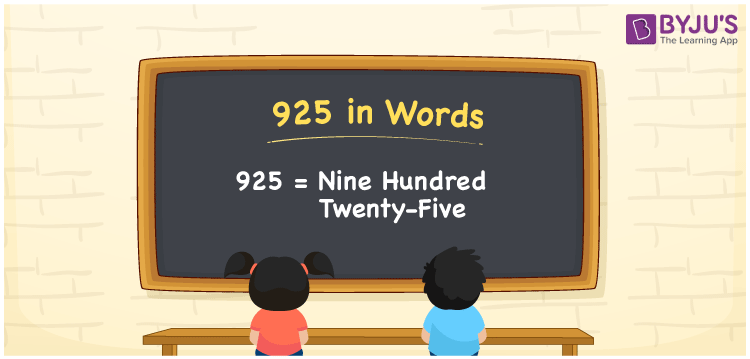# 925 in words

925 in words is written as Nine Hundred and Twenty Five. 925 represents the count or value. The article on Counting Numbers can give you an idea about count or counting. The number 925 is a 3 digit number that is used in expressions related to money, days, distance, length, weight and so on. Let us consider an example for 925. “The letter “a” was used as an article Nine Hundred and Twenty Five times in a document.” Another example is “I need Nine Hundred and Twenty Five Rupees to buy a bag”.

 925 in words Nine Hundred and Twenty Five Nine Hundred and Twenty Five in Numbers 925

## 925 in English Words## How to Write 925 in Words?

We can convert 925 to words using a place value chart. The number 925 has 3 digits, so let’s make a chart that shows the place value up to 3 digits.

 Hundreds Tens Ones 9 2 5

Thus, we can write the expanded form as:

9 × Hundred + 2 × Ten + 5 × One

= 9 × 100 + 2 × 10 + 5 × 1

= 925

= Nine Hundred and Twenty Five.

925 is the natural number that is succeeded by 924 and preceded by 926.

925 in words – Nine Hundred and Twenty Five.

Is 925 an odd number? – Yes.

Is 925 an even number? – No.

Is 925 a perfect square number? – No.

Is 925 a perfect cube number? – No.

Is 925 a prime number? – No.

Is 925 a composite number? – Yes.

## Solved Example

1. Write the number 925 in expanded form

Solution: 9 × 100 + 2 × 10 + 5 × 1

We can write 925 = 900 + 20 + 5

= 9 × 100 + 2 × 10 + 5 × 1.

## Frequently Asked Questions on 925 in words

### How to write the number 925 in words?

925 in words is written as Nine Hundred and Twenty Five.

### State True or False. 925 is divisible by 3?

False. 925 is not divisible by 3.

### Is 925 a perfect square number?

No. 925 is not a perfect square number.